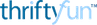# Multiplying By 11

### Tip: Multiplying By 11

The easy way to multiply by eleven when they're learning their times tables!

If you want to multiply 43 x 11
Split the 43 with a space in the middle as: 4 3

Now add the 4 and 3 together (4+3) = 7
Next put the 7 in the middle of the 4 and 3 like this: 473
So, 25 x 11 = 275 and 31 x 11 = 341

Source: Thanks to my junior school teacher of 60+ years agoSilver Post Medal for All Time! 418 Posts
May 24, 20210 found this helpful

That is so neat. It really works.

Categories
PagesMore
Categories